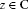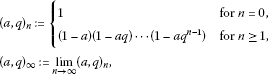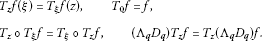Theory and Modern Applications

# Basic Fourier transform on the space of entire functions of logarithm order 2

## Abstract

We study in this paper the basic Fourier transform, q-translation and q-convolution associated to the q-difference operator in the space of entire functions with logarithmic order 2 and finite logarithmic type and their dual.

## 1 Introduction

The concept of the basic Fourier transform is related to the quantum group which is a q-deformation of the Lie group. The deformation parameter q is always assumed to satisfy $0. The basic Fourier transform was defined firstly in  and studied after that from the point of view of harmonic analysis in , ….

In this work, we are interested in the basic Fourier transforms of entire functions with logarithmic order 2 and finite logarithmic type which is introduced by . This notion of logarithmic order is a refinement order of entire functions of order zero which is used to study the growth of order of some basic hypergeometric series. Our investigation is inspired by the ideas developed by [9, 10] and . Some of the arguments used here are similar to the one considered in  and . However, we need to introduce new procedures to prove the results in the q-theory setting.

The paper is organized as follows. In Section 2, we give a brief introduction and recall some known results about q-shift factorial, q-derivative and q-exponential function. In Section 3, firstly we describe the space of entire functions and its dual. Also, we give a new q-Taylor expansion of an entire function. Secondly, we introduce the logarithmic order and logarithmic type. In Section 4, we study a new q-translation operator and its related q-convolution and we give several characterizations from the space of entire functions into itself that commute with the q-translation. Finally, in Section 5, we define the q-Fourier transform on the dual space of entire functions and we establish a q-Paley-Wiener theorem type.

## 2 Preliminaries

We assume thatand $0, unless specified otherwise. We recall some notations . For an arbitrary complex number a,and

${\left[\begin{array}{c}n\\ k\end{array}\right]}_{q}:=\frac{{\left(q,q\right)}_{n}}{{\left(q,q\right)}_{k}{\left(q,q\right)}_{n-k}}.$

The q-derivative of a function $f\left(z\right)$ is defined as in  by

${D}_{q}f\left(z\right):=\frac{f\left(z\right)-f\left(qz\right)}{\left(1-q\right)z},\phantom{\rule{1em}{0ex}}z\ne 0,$

and we say that f has the q-derivative at zero if the limit

$\underset{n\to \mathrm{\infty }}{lim}\frac{f\left(z{q}^{n}\right)-f\left(0\right)}{z{q}^{n}}$

exists and does not depend on z. We define the operator

${\mathrm{\Lambda }}_{q}f\left(z\right):=f\left({q}^{-1}z\right).$

Obviously,

${\mathrm{\Lambda }}_{q}{D}_{q}=q{D}_{q}{\mathrm{\Lambda }}_{q}$

and for an arbitrary positive integer n,

(1)

Consider the q-exponentials (see ) defined by

${e}_{q}\left(z\right):=\sum _{n=0}^{\mathrm{\infty }}\frac{{z}^{n}}{{\left(q,q\right)}_{n}}=\frac{1}{{\left(z,q\right)}_{\mathrm{\infty }}},\phantom{\rule{1em}{0ex}}|z|<1$

and

${E}_{q}\left(z\right):=\sum _{n=0}^{\mathrm{\infty }}\frac{{q}^{\left(\begin{array}{c}n\\ 2\end{array}\right)}{z}^{n}}{{\left(q,q\right)}_{n}}={\left(-z,q\right)}_{\mathrm{\infty }}.$
(2)

The q-exponentials functions ${e}_{q}\left(z\right)$ and ${E}_{q}\left(z\right)$ satisfy

${D}_{q}{e}_{q}\left(z\right)={e}_{q}\left(z\right)\phantom{\rule{1em}{0ex}}\text{and}\phantom{\rule{1em}{0ex}}{\mathrm{\Lambda }}_{q}{D}_{q}{E}_{q}\left(z\right)={E}_{q}\left(z\right).$

## 3 Space of entire functions of finite logarithmic order

We denote by $\mathcal{A}$ the space of entire functions on . This space is endowed with the topology of the uniform convergence on compact subsets of . Thus, $\mathcal{A}$ is a Frechet space. ${\mathcal{A}}^{\mathrm{\prime }}$ denotes the strong dual space of $\mathcal{A}$. If $T\in {\mathcal{A}}^{\mathrm{\prime }}$, there exist a constant $C>0$ and a compact subset K of such that

$|〈T,f〉|\le C\underset{z\in K}{sup}|f\left(z\right)|,\phantom{\rule{1em}{0ex}}f\in \mathcal{A}.$

According to the Hahn-Banach theorem, the mapping T can be extended to the space $C\left(K\right)$ of continuous functions on K, as an element of the dual space of $C\left(K\right)$. Then the Riesz representation theorem implies there exists a regular complex Borel measure γ supported in K such that

$〈T,f〉={\int }_{\mathbb{C}}f\left(z\right)\phantom{\rule{0.2em}{0ex}}d\gamma \left(z\right),\phantom{\rule{1em}{0ex}}f\in \mathcal{A}.$

Proposition 1 The operator${\mathrm{\Lambda }}_{q}{D}_{q}$is continuous from$\mathcal{A}$into its self.

Proof According to the Cauchy integral formula, for $|z|<{q}^{2}r$, we can write

${\mathrm{\Lambda }}_{q}{D}_{q}f\left(z\right)=\frac{1}{2i\pi }{\int }_{|u|=r}\frac{f\left(u\right)}{\left(u-{q}^{-1}z\right)\left(u-z\right)}\phantom{\rule{0.2em}{0ex}}du.$

Then

${|{\mathrm{\Lambda }}_{q}{D}_{q}f|}_{{q}^{2}r}\le \frac{1}{\left(1-q\right)\left(1-{q}^{2}\right)r}{|f|}_{r},$

where ${|f|}_{r}={sup}_{|z|\le r}|f\left(z\right)|$. Thus, we conclude that the operator ${\mathrm{\Lambda }}_{q}{D}_{q}$ is continuous from $\mathcal{A}$ into itself. □

In the following theorem, we establish a new q-Taylor formula different from the one considered in  and .

Theorem 2 If$f\in \mathcal{A}$, then f admits the representation

$f\left(z\right)=\sum _{n=0}^{\mathrm{\infty }}\frac{{\left(1-q\right)}^{n}{q}^{\left(\genfrac{}{}{0}{}{n}{2}\right)}{z}^{n}}{{\left(q;q\right)}_{n}}{\left({\mathrm{\Lambda }}_{q}{D}_{q}\right)}^{n}f\left(0\right).$

Proof Suppose that

$f\left(z\right)=\sum _{n=0}^{\mathrm{\infty }}{a}_{n}{z}^{n}.$

Then for $k=0,1,\dots$ , we have

$\frac{{\left(1-q\right)}^{k}}{{\left(q;q\right)}_{k}}{\left({\mathrm{\Lambda }}_{q}{D}_{q}\right)}^{k}f\left(z\right)=\sum _{n=k}^{\mathrm{\infty }}{a}_{n}{\left[\begin{array}{c}n\\ k\end{array}\right]}_{q}{q}^{\left(\begin{array}{c}k+1\\ 2\end{array}\right)-nk}{z}^{n-k}.$
(3)

By taking $z=0$, we obtain for $k=0,1,\dots$ that

${a}_{k}=\frac{{\left(1-q\right)}^{k}}{{\left(q;q\right)}_{k}}{q}^{\left(\begin{array}{c}k\\ 2\end{array}\right)}{\left({\mathrm{\Lambda }}_{q}{D}_{q}\right)}^{k}f\left(0\right),$
(4)

This proves the result. □

Recent research concerning q-difference equations, moment problems (see [8, 1620]) strongly suggests that in order to deal with basic hypergeometric functions, one should use the following concept.

We recall (see ) that an entire function f of order zero has logarithmic order ϱ if

$\varrho :=\underset{r\to +\mathrm{\infty }}{lim}sup\frac{lnln{|f|}_{r}}{lnlnr},$
(5)

and when the logarithmic order ϱ of f is finite, we define the logarithmic type τ as

$\tau :=\underset{r\to +\mathrm{\infty }}{lim}sup\frac{ln{|f|}_{r}}{{\left(lnr\right)}^{\varrho }}.$
(6)

It is easy to see that:

f is of logarithmic order ρ if and only if, for every $\epsilon >0$,

${|f|}_{r}=O\left({e}^{{\left(lnr\right)}^{\rho +\epsilon }}\right).$

If f is of logarithmic order ρ, then its logarithmic type is equal to τ if and only if

${|f|}_{r}=O\left({e}^{\left(\tau +\epsilon \right){\left(lnr\right)}^{\rho }\right)}\right).$

We denote by ${E}_{\tau ,q}\left(\mathbb{C}\right)$ the space of entire functions of logarithmic order 2 and logarithmic type τ. In particular, when $\tau =\frac{1}{2ln{q}^{-1}}$, ${E}_{\tau ,q}\left(\mathbb{C}\right)$ is denoted simply by ${E}_{q}\left(\mathbb{C}\right)$. That is, an entire function f is in ${E}_{\tau ,q}\left(\mathbb{C}\right)$ if and only if for every $\epsilon >0$,

The space ${E}_{\tau ,q}\left(\mathbb{C}\right)$ is endowed by the topology associated to the family $\left\{{\rho }_{\tau ,\epsilon }\right\}$ of seminorms, where for every $\epsilon >0$,

${\rho }_{\tau ,\epsilon }\left(f\right)=\underset{z\in \mathbb{C}}{sup}|f\left(z\right){e}^{-\left(\tau +\epsilon \right){ln}^{2}|z|}|.$

Thus, ${E}_{\tau ,q}\left(\mathbb{C}\right)$ is a Banach space. The dual of ${E}_{\tau ,q}\left(\mathbb{C}\right)$ is denoted, as usual, by ${E}_{\tau ,q}^{\prime }\left(\mathbb{C}\right)$.

Lemma 3 ()

Let$f\left(z\right)={\sum }_{n=0}^{\mathrm{\infty }}{a}_{n}{z}^{n}$be an entire function of order 0. Its logarithmic order ρ and logarithmic type τ satisfy

$\rho =1+\underset{n\to \mathrm{\infty }}{lim sup}\frac{lnn}{lnln\left(\frac{1}{\sqrt[n]{|{a}_{n}|}}\right)}$
(7)

and

$\tau =\frac{{\left(\rho -1\right)}^{\rho -1}}{{\rho }^{\rho }}\underset{n\to \mathrm{\infty }}{lim sup}\frac{n}{{\left(ln\left(\frac{1}{\sqrt[n]{|{a}_{n}|}}\right)\right)}^{\rho -1}}.$
(8)

Proposition 4 The logarithmic order of the q-exponential function${E}_{q}\left(z\right)$is equal to 2 and its logarithmic type is$\frac{1}{-2ln\left(q\right)}$.

Proof The result follows from Lemma 3. □

Proposition 5 Let χ be a functional on${E}_{\tau ,q}\left(\mathbb{C}\right)$, then$\chi \in {E}_{\tau ,q}^{\prime }\left(\mathbb{C}\right)$if and only if there exists a Borel measure γ on and$\epsilon >0$such that for every$f\in {E}_{\tau ,q}\left(\mathbb{C}\right)$,

$〈\chi ,f〉={\int }_{\mathbb{C}}f\left(z\right){e}^{-\left(\tau +\epsilon \right){\left(ln|z|\right)}^{2}}\phantom{\rule{0.2em}{0ex}}d\gamma \left(z\right).$
(9)

Proof Suppose that χ admits the representation (9) for a certain complex regular Borel measure γ on and $\epsilon >0$. Then we have

$|〈\chi ,f〉|=C|f\left(z\right)|\underset{z}{sup}{e}^{-\left(\tau +\epsilon \right){\left(ln|z|\right)}^{2}}.$

Then $\chi \in {E}_{\tau ,q}^{\prime }\left(\mathbb{C}\right)$. Conversely, let $\chi \in {E}_{\tau ,q}^{\prime }\left(\mathbb{C}\right)$, then there exists C such that

$|〈\chi ,f〉|=C|f\left(z\right)|\underset{z}{sup}{e}^{-\left(\tau +\epsilon \right){\left(ln|z|\right)}^{2}}.$

We denote by ${C}_{0}$ the space of continuous functions in vanishing at infinity. If $f\in {E}_{\tau ,q}\left(\mathbb{C}\right)$, then $f\left(z\right){e}^{-\left(\tau +\epsilon \right){\left(ln|z|\right)}^{2}}\in {C}_{0}$. Indeed for $0<\eta <\epsilon$, we have

$|f\left(z\right)|{e}^{-\left(\tau +\epsilon \right){\left(ln|z|\right)}^{2}}\le \underset{z}{sup}\left(|f\left(z\right)|{e}^{-\left(\tau +\eta \right){\left(ln|z|\right)}^{2}}\right){e}^{-\left(\epsilon -\eta \right){\left(ln|z|\right)}^{2}}\to 0,$

as $|z|\to \mathrm{\infty }$.

We consider the mapping I from ${E}_{\tau ,q}\left(\mathbb{C}\right)$ into ${C}_{0}$ defined by $I\left(f\right)\left(z\right)=f\left(z\right){e}^{-\left(\tau +\epsilon \right){\left(ln|z|\right)}^{2}}$ and the functional λ from $I\left({E}_{\tau ,q}\left(\mathbb{C}\right)\right)$ into given by $\lambda \left(f\right)=〈\chi ,f〉$.

It is clear that λ is continuous when on $I\left({E}_{\tau ,q}\left(\mathbb{C}\right)\right)$ we consider the topology induced in it by the usual topology of ${C}_{0}$. By using Hanh-Banach and Riesz representation theorems in a standard way, we can conclude that χ admits a representation like (9) for a certain complex regular Borel measure γ on and $\epsilon >0$. □

## 4 q-translation and q-convolution

In this section, we define a new q-translation operator related to q-difference operator ${\mathrm{\Lambda }}_{q}{D}_{q}$, on the space of entire functions of logarithm order 2.

Definition 6 The q-translation ${T}_{\xi }$ is defined on monomials ${z}^{n}$ by

${T}_{\xi }{z}^{n}:=\sum _{k=0}^{n}{\left[\begin{array}{c}n\\ k\end{array}\right]}_{q}{q}^{-\left(n-k\right)k}\frac{{\left(1-q\right)}^{k}}{{\left(q;q\right)}_{k}}{\xi }^{k}{z}^{n-k}.$
(10)

Proposition 7 For$\xi \in \mathbb{C}$, the q-translation operator${T}_{\xi }$can be extended on the entire function$f\left(z\right)={\sum }_{n=0}^{\mathrm{\infty }}{a}_{n}{z}^{n}$of logarithmic order 2 and logarithmic type lesser than$\frac{1}{-2lnq}$in the following manner:

${T}_{\xi }f\left(z\right)=\sum _{n=0}^{\mathrm{\infty }}{a}_{n}\sum _{k=0}^{n}{\left[\begin{array}{c}n\\ k\end{array}\right]}_{q}\frac{{\left(1-q\right)}^{k}}{{\left(q;q\right)}_{k}}{q}^{-\left(n-k\right)k}{\xi }^{k}{z}^{n-k}.$
(11)

Proof It suffices to prove the convergence of the following series:

$A=\sum _{n=0}^{\mathrm{\infty }}|{a}_{n}|\sum _{k=0}^{n}{\left[\begin{array}{c}n\\ k\end{array}\right]}_{q}\frac{{\left(1-q\right)}^{k}}{{\left(q;q\right)}_{k}}{q}^{-\left(n-k\right)k}{|\xi |}^{k}{|z|}^{n-k}.$

For every $n\in \mathbb{N}$ and $k=0,\dots ,n$, we can write

$-\left(n-k\right)k=\frac{k\left(k-1\right)}{2}+\frac{1}{2}{k}^{2}+\left(\frac{1}{2}-n\right)k.$

On the other hand, the function

$\phi \left(x\right)=\frac{1}{2}{x}^{2}+\left(\frac{1}{2}-n\right)x$

attains its minimum on $n-\frac{1}{2}$. For such a value $n-\frac{1}{2}$, the minimum value over $\left(0,\mathrm{\infty }\right)$ is

$-\frac{1}{2}{\left(n-\frac{1}{2}\right)}^{2}.$

Hence,

${q}^{-\left(n-k\right)k}\le {q}^{\left(\begin{array}{c}k\\ 2\end{array}\right)}{q}^{-\frac{1}{2}{\left(n-\frac{1}{2}\right)}^{2}}.$
(12)

Furthermore,

$\frac{{\left(1-q\right)}^{k}}{{\left(q;q\right)}_{k}}\le 1.$

Thus,

$A\le \sum _{n=0}^{\mathrm{\infty }}|{a}_{n}|{q}^{-\frac{1}{2}{\left(n-\frac{1}{2}\right)}^{2}}\sum _{k=0}^{n}{\left[\begin{array}{c}n\\ k\end{array}\right]}_{q}{q}^{\left(\begin{array}{c}k\\ 2\end{array}\right)}{|\xi |}^{k}{|z|}^{n-k}.$
(13)

By q-binomial theorem, we get

$\sum _{k=0}^{n}{\left[\begin{array}{c}n\\ k\end{array}\right]}_{q}{q}^{\left(\begin{array}{c}k\\ 2\end{array}\right)}{|\xi |}^{k}{|z|}^{n-k}={|z|}^{n}{\left(-\frac{\xi }{|z|};q\right)}_{n}.$
(14)

Hence,

$|A|\le \sum _{n=0}^{\mathrm{\infty }}|{a}_{n}|{q}^{-\frac{1}{2}{\left(n-\frac{1}{2}\right)}^{2}}{|z|}^{n}{\left(-\frac{|\xi |}{|z|};q\right)}_{n}.$
(15)

The logarithmic type τ of the function f is lesser than $\frac{1}{-2lnq}$, then there exists $0<\beta <1$ such that $\tau =\beta \frac{1}{-2lnq}$. By applying Cauchy estimates, we find, for every $R>0$ and $n\in \mathbb{N}$,

$|{a}_{n}|\le C{R}^{-n}{e}^{\beta \frac{{\left(lnR\right)}^{2}}{2ln{q}^{-1}}}.$
(16)

In particular, for $R={q}^{-n}$, we get

$|{a}_{n}|\le C{q}^{\left(1-\frac{1}{2}\beta \right){n}^{2}}.$

Then

$|A|\le C\sum _{n=0}^{\mathrm{\infty }}{q}^{\frac{1}{2}\left(1-\beta \right){n}^{2}}{q}^{n+\frac{1}{4}}|{z}^{n}|{\left(-\frac{|\xi |}{|z|};q\right)}_{n}<\mathrm{\infty }.$

This shows the result. □

Proposition 8 For$z,\xi \in \mathbb{C}$, the q-exponential function satisfies the product formula

${T}_{\xi }{E}_{q}\left(z\right)={E}_{q}\left(z\right){E}_{q}\left(\xi \right).$

Proof Let f be an entire function of logarithmic order 2 and logarithmic type lesser than $\frac{1}{-2lnq}$. The series defined in (11) is absolutely convergent. Then, after interchanging the double sum, we can write

${T}_{\xi }f\left(z\right)=\sum _{k=0}^{\mathrm{\infty }}\frac{{\left(1-q\right)}^{k}}{{\left(q;q\right)}_{k}}{q}^{-\left(n-k\right)k}{q}^{\left(\genfrac{}{}{0}{}{k}{2}\right)}{\xi }^{k}\sum _{n=k}^{\mathrm{\infty }}{a}_{n}{\left[\begin{array}{c}n\\ k\end{array}\right]}_{q}{q}^{\left(\genfrac{}{}{0}{}{k+1}{2}\right)-nk}{z}^{n-k}.$

By (3), the operator ${T}_{\xi }$ can be represented by the form

${T}_{\xi }f\left(z\right)=\sum _{n=0}^{\mathrm{\infty }}\frac{{\left(1-q\right)}^{n}}{{\left(q;q\right)}_{n}}{q}^{\left(\begin{array}{c}n\\ 2\end{array}\right)}{\xi }^{n}{\left({\mathrm{\Lambda }}_{q}{D}_{q}\right)}^{n}\left(f\right)\left(z\right).$
(17)

The result follows from the fact that the q-exponential function ${E}_{q}\left(z\right)$ is an eigenfunction of the operator ${\mathrm{\Lambda }}_{q}{D}_{q}$ corresponding to the eigenvalue 1. □

Proposition 9 For$f\in {E}_{\tau ,q}\left(\mathbb{C}\right)$, with τ lesser than$\frac{1}{-2lnq}$and$z,\xi \in \mathbb{C}$, we haveNow, we define the q-convolution of $\chi \in {E}_{\tau ,q}^{\prime }\left(\mathbb{C}\right)$ and $f\in {E}_{\tau ,q}\left(\mathbb{C}\right)$ as

$\chi \star f\left(\xi \right)=〈\chi ;{T}_{\xi }f〉.$
(18)

In the following theorem, we obtain several characterizations of the continuous linear mappings L from $\mathcal{A}$ into itself that commute with the q-translation operators.

Theorem 10 Let L be a continuous linear mapping from$\mathcal{A}$into itself. The following assertions are equivalent:

1. (i)

L commutes with the q-translation operators ${T}_{z}$, $z\in \mathbb{C}$, that is,

${T}_{z}L=L{T}_{z},\phantom{\rule{1em}{0ex}}z\in \mathbb{C}.$
2. (ii)

L commutes with the q-difference operator ${\mathrm{\Lambda }}_{q}{D}_{q}$.

3. (iii)

There exists a unique $\vartheta \in {\mathcal{A}}^{\mathrm{\prime }}$ such that $Lf=\vartheta \ast f$, $f\in \mathcal{A}$.

4. (iv)

There exists a complex Borel regular measure having compact support on , for which, for all $f\in \mathcal{A}$, we have

$L\left(f\right)\left(z\right)={\int }_{\mathbb{C}}{\sigma }_{z}f\left(w\right)\phantom{\rule{0.2em}{0ex}}d\gamma \left(w\right).$
5. (v)

There exists an entire function Ψ of logarithmic order 2 such that

$Lf\left(z\right)=\mathrm{\Psi }\left({\mathrm{\Lambda }}_{q}{D}_{q}\right)f\left(z\right),$

where

$\mathrm{\Psi }\left(z\right)=\sum _{n=0}^{\mathrm{\infty }}{a}_{n}{z}^{n}.$

Proof (i) (ii) Let $g\in \mathcal{A}$, we have

$\frac{{T}_{z}g\left(\xi \right)-g\left(\xi \right)}{z}=\sum _{n=1}^{\mathrm{\infty }}\frac{{\left(1-q\right)}^{n}}{{\left(q;q\right)}_{n}}{q}^{\left(\begin{array}{c}n\\ 2\end{array}\right)}{z}^{n-1}{\left({\mathrm{\Lambda }}_{q}{D}_{q}\right)}^{n}\left(g\right)\left(\xi \right).$
(19)

Hence,

$\underset{z\to 0}{lim}\frac{{T}_{z}g\left(\xi \right)-g\left(\xi \right)}{z}={\mathrm{\Lambda }}_{q}{D}_{q}g\left(\xi \right).$

The operator L is continuous, then

${\mathrm{\Lambda }}_{q}{D}_{q}Lg\left(\xi \right)=\underset{z\to 0}{lim}\frac{{T}_{z}Lg\left(\xi \right)-Lg\left(\xi \right)}{z}=L\underset{z\to 0}{lim}\frac{{T}_{z}g\left(\xi \right)-g\left(\xi \right)}{z}=L{\mathrm{\Lambda }}_{q}{D}_{q}g\left(\xi \right).$

Hence, (i) (ii).

(ii) (i) The operator L commutes with ${\mathrm{\Lambda }}_{q}{D}_{q}$, then for every $n\in \mathbb{N}$,

$L{\left({\mathrm{\Lambda }}_{q}{D}_{q}\right)}^{n}={\left({\mathrm{\Lambda }}_{q}{D}_{q}\right)}^{n}L.$

Hence,

$\begin{array}{rcl}L{T}_{z}g\left(\xi \right)& =& \sum _{n=1}^{\mathrm{\infty }}\frac{{\left(1-q\right)}^{n}}{{\left(q;q\right)}_{n}}{q}^{\left(\begin{array}{c}n\\ 2\end{array}\right)}{z}^{n-1}L{\left({\mathrm{\Lambda }}_{q}{D}_{q}\right)}^{n}\left(g\right)\left(\xi \right)\\ =& \sum _{n=1}^{\mathrm{\infty }}\frac{{\left(1-q\right)}^{n}}{{\left(q;q\right)}_{n}}{q}^{\left(\begin{array}{c}n\\ 2\end{array}\right)}{z}^{n-1}{\left({\mathrm{\Lambda }}_{q}{D}_{q}\right)}^{n}L\left(g\right)\left(\xi \right)\\ =& {T}_{z}Lg\left(z\right).\end{array}$
1. (i)

(iii) Assume that (i) holds. We define the functional ϑ on $\mathcal{A}$ as follows:

$〈\vartheta ;f〉=Lf\left(0\right).$

It is clear that $\vartheta \in {\mathcal{A}}^{\prime }$ and

$\vartheta \star f\left(\xi \right)=〈\vartheta ;{T}_{\xi }f〉=L{T}_{\xi }f\left(0\right)=Lf\left(\xi \right).$
1. (iii)

(iv) It follows immediately from Hahn-Banach and Riesz representation theorems.

2. (iv)

(v) Suppose that for all f, we have

$Lf\left(\xi \right)={\int }_{\mathbb{C}}{T}_{\xi }f\left(z\right)\phantom{\rule{0.2em}{0ex}}d\gamma \left(z\right),$

where γ is a complex Borel regular measure with compact support. According to , we obtain

$L\left(f\right)\left(z\right)=\sum _{n=0}^{\mathrm{\infty }}{\left({\mathrm{\Lambda }}_{q}{D}_{q}\right)}^{n}f\left(z\right){\int }_{\mathbb{C}}\frac{{\left(1-q\right)}^{n}}{{\left(q;q\right)}_{n}}{q}^{\left(\begin{array}{c}n\\ 2\end{array}\right)}{\xi }^{n}\phantom{\rule{0.2em}{0ex}}d\gamma \left(\xi \right).$

Hence,

$Lf\left(z\right)=\mathrm{\Psi }\left({\mathrm{\Lambda }}_{q}{D}_{q}\right)f\left(z\right),$

where

Since γ has compact support on , for certain a and C, we have

$|{a}_{n}|\le \frac{{\left(1-q\right)}^{n}}{{\left(q;q\right)}_{n}}{q}^{\left(\begin{array}{c}n\\ 2\end{array}\right)}{a}^{n}.$

Then we get

$|\mathrm{\Psi }\left(z\right)|\le {E}_{q}\left(a|z|\right),$

and Ψ is of logarithmic order 2.

1. (iv)

(i) Suppose that for every $f\in \mathcal{A}$, we obtain

$L\left(f\right)\left(z\right)=\sum _{n=0}^{\mathrm{\infty }}{\left({\mathrm{\Lambda }}_{q}{D}_{q}\right)}^{n}f\left(z\right){\int }_{\mathbb{C}}\frac{{\left(1-q\right)}^{n}}{{\left(q;q\right)}_{n}}{q}^{\left(\begin{array}{c}n\\ 2\end{array}\right)}{\xi }^{n}\phantom{\rule{0.2em}{0ex}}d\gamma \left(\xi \right).$

Hence, if $f\in \mathcal{A}$ since ${T}_{z}{\mathrm{\Lambda }}_{q}{D}_{q}f={\mathrm{\Lambda }}_{q}{D}_{q}{T}_{z}f$,

$\begin{array}{rcl}{T}_{z}L\left(f\right)\left(z\right)& =& \sum _{n=0}^{\mathrm{\infty }}{T}_{z}{\left({\mathrm{\Lambda }}_{q}{D}_{q}\right)}^{n}f\left(z\right)\\ =& \sum _{n=0}^{\mathrm{\infty }}{\left({\mathrm{\Lambda }}_{q}{D}_{q}\right)}^{n}{T}_{z}f\left(z\right)\\ =& L{T}_{z}f.\end{array}$

□

## 5 The q-Fourier transform on the space ${\mathcal{A}}^{\prime }$

We introduce the q-Fourier transform on ${\mathcal{A}}^{\mathrm{\prime }}$ by

${\mathcal{F}}_{q}\left(T\right)\left(\xi \right)=〈T\left(z\right),{E}_{q}\left(-i\xi z\right)〉,\phantom{\rule{1em}{0ex}}\xi ,z\in \mathbb{C}.$
(20)

From (2) the q-Fourier transform takes the form

${\mathcal{F}}_{q}\left(T\right)\left(\xi \right)=\sum _{n=0}^{\mathrm{\infty }}{\left(-i\right)}^{n}\frac{{\left(1-q\right)}^{n}{q}^{\left(\genfrac{}{}{0}{}{n}{2}\right)}}{{\left(q;q\right)}_{n}}〈T,{z}^{n}〉{\xi }^{n},\phantom{\rule{1em}{0ex}}\xi \in \mathbb{C}.$
(21)

Theorem 11 (q-Paley-Wiener theorem)

The q-Fourier transform${\mathcal{F}}_{q}$is a topological isomorphism from${\mathcal{A}}^{\prime }$onto${E}_{q}\left(\mathbb{C}\right)$.

Proof Assume firstly that $T\in {\mathcal{A}}^{\prime }$. Then there exists a complex regular Borel measure γ on and a > 0 such that the support of it is contained in the open ball $B\left(0,a\right)$ of center 0 and radius a and

$〈T,f〉={\int }_{\mathbb{C}}f\left(z\right)\phantom{\rule{0.2em}{0ex}}d\gamma \left(z\right),\phantom{\rule{1em}{0ex}}f\in \mathcal{A}$

so that for every $n\in \mathbb{N}$, there exists a constant $C>0$ such that

$|〈T;{z}^{n}〉|\le C{a}^{n}.$
(22)

By the expansion (21) of the q-Fourier of T, we can write

${\mathcal{F}}_{q}\left(T\right)\left(\lambda \right)={\int }_{\mathbb{C}}{E}_{q}\left(-i\lambda z\right)\phantom{\rule{0.2em}{0ex}}d\gamma \left(z\right)=\sum _{n=0}^{\mathrm{\infty }}{a}_{n},$

where

${a}_{n}={\left(-i\right)}^{n}\frac{{\left(1-q\right)}^{n}{q}^{\left(\genfrac{}{}{0}{}{n}{2}\right)}}{{\left(q;q\right)}_{n}}〈T,{z}^{n}〉{\lambda }^{n},\phantom{\rule{1em}{0ex}}\lambda \in \mathbb{C}.$

Then

$\frac{1}{n}ln|{a}_{n}|=ln\left(1-q\right)+ln|\lambda |-ln{\left(q;q\right)}_{n}+ln|〈T;{z}^{n}〉|+\frac{n-1}{2}ln\left(q\right)$

and

$\begin{array}{rcl}lnln\left(\frac{1}{\sqrt[n]{|{a}_{n}|}}\right)& =& ln\left(n-1\right)+ln\left(-\frac{ln\left(q\right)}{2}\right)+ln\left[1-\frac{2ln\left({\left(q;q\right)}_{n}\right)}{n\left(n-1\right)ln\left(q\right)}\\ +2\frac{ln\left(1-q\right)+ln|\lambda |}{\left(n-1\right)ln\left(q\right)}+2\frac{ln|〈T;{z}^{n}〉|}{n\left(n-1\right)ln\left(q\right)}\right].\end{array}$

The inequality (22) shows that

$\underset{n\to \mathrm{\infty }}{lim}\frac{ln|〈T;{z}^{n}〉|}{n\left(n-1\right)ln\left(q\right)}=0.$

Then

$\underset{n\to \mathrm{\infty }}{lim}\frac{lnln\left(\frac{1}{\sqrt[n]{|{a}_{n}|}}\right)}{lnn}=1.$

Furthermore, the logarithmic order ρ of ${\mathcal{F}}_{q}\left(T\right)$ is equal to

$\rho =1+\underset{n\to \mathrm{\infty }}{lim sup}\frac{lnn}{lnln\left(\frac{1}{\sqrt[n]{|{a}_{n}|}}\right)}=2.$

Similarly, the logarithmic type of τ of ${\mathcal{F}}_{q}\left(T\right)$ is given by

$\tau =\underset{n\to \mathrm{\infty }}{lim sup}\frac{n}{ln\left(\frac{1}{\sqrt[n]{|{a}_{n}|}}\right)}=\frac{1}{-2lnq}.$

Hence, we have proved ${\mathcal{F}}_{q}\left(T\right)\in {E}_{q}\left(\mathbb{C}\right)$. Moreover, ${\mathcal{F}}_{q}$ is a continuous mapping from $\mathcal{A}$ into ${E}_{q}\left(\mathbb{C}\right)$. Indeed, since $\mathcal{A}$ is a bornological space, it is sufficient to see that ${\mathcal{F}}_{q}\left(T\right)\in {E}_{q}\left(\mathbb{C}\right)$. The q-Fourier transform ${\mathcal{F}}_{q}$ is a one-to-one mapping from ${\mathcal{A}}^{\prime }$ into ${E}_{q}\left(\mathbb{C}\right)$. Indeed, assume that $T\in {\mathcal{A}}^{\prime }$ is such that ${\mathcal{F}}_{q}\left(T\right)=0$, $z\in \mathbb{C}$. Then from (20), we infer that

$〈T\left(w\right),{w}^{n}〉=0,\phantom{\rule{1em}{0ex}}\mathrm{\forall }n\in \mathbb{N}.$

Hence, (21) implies also that

$〈T\left(w\right),f〉=0,\phantom{\rule{1em}{0ex}}\mathrm{\forall }f\in \mathcal{A}.$

Thus, the transform ${\mathcal{F}}_{q}$ is one-to-one. Suppose now that g is a function in ${E}_{q}\left(\mathbb{C}\right)$. There exists $C>0$ such that for every $r>0$,

$|g{|}_{r}\le C{e}^{\frac{{ln}^{2}|r|}{2ln{q}^{-1}}}.$

From Theorem 2, we have

$g\left(z\right)=\sum _{n=0}^{\mathrm{\infty }}\frac{{\left(1-q\right)}^{n}{q}^{\left(\genfrac{}{}{0}{}{n}{2}\right)}{z}^{n}}{{\left(q;q\right)}_{n}}{\left({\mathrm{\Lambda }}_{q}{D}_{q}\right)}^{n}g\left(0\right),\phantom{\rule{1em}{0ex}}z\in \mathbb{C}.$

Then, for every $n\in \mathbb{N}$,

$\frac{{\left(1-q\right)}^{n}{q}^{\left(\genfrac{}{}{0}{}{n}{2}\right)}}{{\left(q;q\right)}_{n}}{\left({\mathrm{\Lambda }}_{q}{D}_{q}\right)}^{n}g\left(0\right)={\int }_{{C}_{R}}\frac{g\left(w\right)}{{w}^{n+1}}\phantom{\rule{0.2em}{0ex}}d\gamma \left(w\right),$

where ${C}_{R}$ represents, for every $R>0$, the circular path ${C}_{R}:w=R{e}^{i\theta }$, $\theta \in \left[0,2\right)$. Hence, for every $n\in \mathbb{N}$, we have

$|{\left({\mathrm{\Lambda }}_{q}{D}_{q}\right)}^{n}g\left(0\right)|\le C\frac{{\left(q;q\right)}_{\mathrm{\infty }}}{{\left(1-q\right)}^{n}{R}^{n}{q}^{\left(\genfrac{}{}{0}{}{n}{2}\right)}}{e}^{\frac{{ln}^{2}|R|}{2ln{q}^{-1}}}\rho \left(g\right).$

In particular, if we take $R={q}^{-n}$, we obtain

$|{\left({\mathrm{\Lambda }}_{q}{D}_{q}\right)}^{n}g\left(0\right)|\le {C}^{\mathrm{\prime }}{a}^{n},\phantom{\rule{1em}{0ex}}a=\frac{{q}^{1/2}}{1-q}.$

On the other hand, from (2), we have

$\frac{1}{2i\pi }{\int }_{{C}_{2a}}{E}_{q}\left(-iz\xi \right){\xi }^{-1-n}\phantom{\rule{0.2em}{0ex}}d\xi =\frac{{\left(1-q\right)}^{n}}{{\left(q;q\right)}_{n}}{q}^{\left(\begin{array}{c}n\\ 2\end{array}\right)}{\left(-iz\right)}^{n}.$

For $|z|>a$, we put

$f\left(z\right)=\sum _{m=0}^{\mathrm{\infty }}{\left(-i\right)}^{-m}{z}^{-1-m}{\left({\mathrm{\Lambda }}_{q}{D}_{q}\right)}^{m}\left(g\right)\left(0\right).$

Then the series converges absolutely in ${C}_{2a}$. Hence, for every $z\in \mathbb{C}$, we have

$\begin{array}{rcl}\frac{1}{2i\pi }{\int }_{{C}_{2a}}{E}_{q}\left(-iz\xi \right)f\left(\xi \right)\phantom{\rule{0.2em}{0ex}}d\xi & =& \sum _{m=0}^{\mathrm{\infty }}{\left(-iz\right)}^{n}{\left({\mathrm{\Lambda }}_{q}{D}_{q}\right)}^{n}\left(g\right)\left(0\right)\frac{1}{2i\pi }{\int }_{{C}_{2a}}{E}_{q}\left(-iz\xi \right){\xi }^{-1-n}\phantom{\rule{0.2em}{0ex}}d\xi \\ =& \sum _{m=0}^{\mathrm{\infty }}\frac{{\left(1-q\right)}^{n}{q}^{\left(\genfrac{}{}{0}{}{n}{2}\right)}}{{\left(q;q\right)}_{n}}{z}^{n}{\left({\mathrm{\Lambda }}_{q}{D}_{q}\right)}^{n}\left(g\right)\left(0\right)\\ =& g\left(z\right).\end{array}$

We now define the functional T on $\mathcal{A}$ by

$〈\chi ;\phi 〉=\frac{1}{2i\pi }{\int }_{{C}_{2a}}f\left(z\right)\phi \left(z\right)\phantom{\rule{0.2em}{0ex}}dz.$

It is obvious that $\chi \in \mathcal{A}$. Moreover, ${\mathcal{F}}_{q}\left(T\right)=g$. Also, from (22), we deduce that for every bounded set Ω of $\mathcal{A}$, there exists $C>0$ such that

$〈{\mathcal{F}}^{-1}\left(g\right);\phi 〉\le C\underset{z\in \mathrm{\Omega }}{sup}|g\left(z\right)|.$
(23)

We conclude that ${\mathcal{F}}^{-1}$ is continuous from ${E}_{q}\left(\mathbb{C}\right)$ onto $\mathcal{A}$. □

## References

1. Kempf A, Majid S: Algebraic q -integration and Fourier theory on quantum and braided spaces. J. Math. Phys. 1994, 35: 6802–6837. 10.1063/1.530644

2. Koornwinder T: Special functions and q -commuting variables. Fields Inst. Commun. 14. In Special Functions, q-Series and Related Topics (Toronto, ON, 1995). Am. Math. Soc., Providence; 1997:131–166. q-alg/9608008

3. Olshanetsky, MA, Rogov, V-BK: The q-Fourier transform of q-distributions. Preprint IHES, 91440-Bures-sur-Yvette (1998)

4. Carnovale G, Koornwinder TH: A q -analogue of convolution on the line. Methods Appl. Anal. 2000, 7: 705–726.

5. Bonet J: Hypercyclic and chaotic convolution operators. J. Lond. Math. Soc. 2000, 62: 252–262.

6. Annaby MH, Hassan HA, Mansour ZS: Sampling theorems associated with singular q -Sturm Liouville problems. Results Math. 2012, 62: 121–136. 10.1007/s00025-011-0134-9

7. Abreu LD: A q -sampling theorem related to the q -Hankel transform. Proc. Am. Math. Soc. 2005, 133: 1197–1203. 10.1090/S0002-9939-04-07589-6

8. Berg C, Pedersen HL, Hayman W: Logarithmic order and type of indeterminate moment problems. In Proceedings of the International Conference ‘Difference Equations, Special Functions and Orthogonal Polynomials’. Edited by: Elaydi S, Cushing J, Lasser R, Papageorgiou V, Ruffing A, Assche W. World Scientific, Singapore; 2007:51–79.

9. Ehrenpreis L: Solution of some problems of division, III. Am. J. Math. 1956, 78: 685–715. 10.2307/2372464

10. Belhadj M, Betancor JJ: Hankel convolution operators on entire functions and distributions. J. Math. Anal. Appl. 2002, 276: 40–63. 10.1016/S0022-247X(02)00350-5

11. Betancor JJ, Betancor JD, M’endez JM: Hypercyclic and chaotic convolution operators in Chebli-Trimeche hypergroups. Rocky Mt. J. Math. 2004, 34: 1207–1238. 10.1216/rmjm/1181069796

12. Gasper G, Rahman M Encyclopedia of Mathematics and Its Applications 96. In Basic Hypergeometric Series. 2nd edition. Cambridge University Press, Cambridge; 2004.

13. Jackson FH: On a q -definite integrals. Q. J. Pure Appl. Math. 1910, 41: 193–203.

14. Ismail MEH, Stanton D: Applications of q -Taylor theorems. J. Comput. Appl. Math. 2003, 153: 259–272. 10.1016/S0377-0427(02)00644-1

15. Ismail MEH, Stanton D: q -Taylor theorems, polynomial expansions, and interpolation of entire functions. J. Approx. Theory 2003, 123: 125–146. 10.1016/S0021-9045(03)00076-5

16. Bouzeffour F: Interpolation of entire functions, product formula for basic sine function. J. Nonlinear Math. Phys. 2006, 13(2):293–301. 10.2991/jnmp.2006.13.2.11

17. Bouzeffour F: A q -sampling theorem and product formula for continuous q -Jacobi functions. Proc. Am. Math. Soc. 2007, 135: 2131–2139. MR 2299491 (2008k:33055) 10.1090/S0002-9939-07-08717-5

18. Bouzeffour F: A Whittaker-Shannon-Kotel’nikov sampling theorem related to the Askey-Wilson functions. J. Nonlinear Math. Phys. 2007, 14(3):367–380. MR 2350096 (2008h:94031)

19. Abreu LD, Bouzeffour F: A Paley-Wiener theorem for the Askey-Wilson function transform. Proc. Am. Math. Soc. 2010, 138: 2853–2862. 10.1090/S0002-9939-10-10327-X

20. Ramis JP: About the growth of entire functions solutions of linear algebraic q -difference equations. Ann. Fac. Sci. Toulouse, Math. 1992, 1(6):53–94.

## Acknowledgements

This research is supported by NPST Program of King Saud University, project number 10-MAT1293-02.

## Author information

Authors

### Corresponding author

Correspondence to F Bouzeffour.

### Competing interests

The author declares that they have no competing interests.

## Rights and permissions

Reprints and Permissions

Bouzeffour, F. Basic Fourier transform on the space of entire functions of logarithm order 2. Adv Differ Equ 2012, 184 (2012). https://doi.org/10.1186/1687-1847-2012-184

• Accepted:

• Published:

• DOI: https://doi.org/10.1186/1687-1847-2012-184

### Keywords

• Entire Function
• Continuous Linear Mapping
• Riesz Representation Theorem
• Cauchy Integral Formula
• Basic Hypergeometric Series